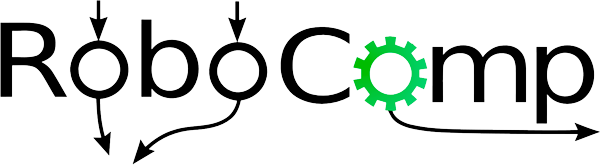# Deep Reinforcement Learning Algorithms

## Approach

The aim of the project is also to implement various deep RL algorithms. A few deep RL algorithms were written. There were two architectures that were implemented. First is a normal MLP based network, wherein the underlying module in each network will be a simple multi-layered-perceptron. The other architecture that we used is a transformer-style network.

1. DQN

The very first network that was written was Deep Q-Learning network. This is the most simple off-policy network that started the break-through in deep RL research. Two important features are the fixed targets, and the replay buffer. The replay buffer is used to store the trajectories observed, and states are sampled from this to update a neural network whose aim is to learn the Q-value function. Fixed targets are used for stabilising the training, and is updated periodically.

2. Dueling DQN

This is a slight modification to the DQN architecture. In this there are two heads, in which one of the head estimates the state-value function, and the other head tries to estimate the advantage function. Advantage function, as the name suggests, gives the difference between Q(s,a) and V(s) [how advatageous is it to take an action]. The rest of the training is similar the DQN network.

3. A2C

The above two methods are ways of estimating the Q value function, and generally an epsilon-greedy policy is applied to learn the policy. A2C (Advantage Actor Critic) is a method that is used to directly learn the policy function, and finally converge to the optimal policy. This is a policy gradient approach, and an on policy algorithm. The explanation behind the algorithm involves a bit of math, but in short, a neural network is learnt that predicts the probabilites of taking actions given the state. Finally it should be able to learn such a policy that the maximum probability should be for the optimal action given the state.

4. PPO

Proximal Policy Optimisation (PPO) is another on-policy algorithm, and is used to estimate the policy function. The problem with on policy methods is that we do not have much data sampled using the current policy, because once we update the network, the policy changes. So, we would want to have data, as well as work on-policy. This is handled using a trick called importance sampling. The other important point regarding PPO, is that there is a clipping that is used instead of a trust region as proposed in an earlier paper (TRPO).

All these algorithms were written, and a few experiments were conducted. A glimpse of the dueling DQN on an environment with just the goal and no obstacles can be seen here:

https://user-images.githubusercontent.com/57453637/185982885-70aed38c-4855-4ed9-a32e-9cfdc5171a88.mp4